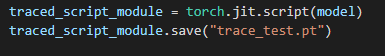# faster-RCNN to libtorch issue

I have finetuned a faster-RCNN model and am trying to shift the code to c++ using the jit.script.

I tried with jit.trace as instructed in the pytorch website. However, there was error. Upon searching in the internet I found the torch vision models are scriptable not traceable (i dont know the difference).

thus I have created a .pt file using the torch.jit.script(model)I setup the c++ environment with libtorch, opencv etc and am trying to run the code. However, i am running with two problem.

problem 1: I trained the model in python with shape (720,1280) and when i run the code on the c++. i received this error…Note: the code runs fine in the python version with the same size

Problem 2:
I then changed the shape of the image (resize) to (275,256) by looking at this example code

Then
I am receiving this output

The prediction is null
(to be sure that the open cv is extracting frames. I saved the frames as video in c++. The output videos were fine.)
Since the predicyion is null, I am doubting that my scripting method might have been wrong in the python.

The c++ code is given below.(writing a code in c++ after a very very very long time so there might be a bug causing the error. Please review it.)

``````int main(){

// Create a VideoCapture object and use camera to capture the video
VideoCapture cap("/workspace/cpp/input.mp4");

torch::jit::script::Module module;
// Default resolutions of the frame are obtained.The default resolutions are system dependent.
int frame_width = cap.get(cv::CAP_PROP_FRAME_WIDTH);
int frame_height = cap.get(cv::CAP_PROP_FRAME_HEIGHT);

// Define the codec and create VideoWriter object.The output is stored in 'outcpp.avi' file.
//  VideoWriter video("outcpp.avi", cv::VideoWriter::fourcc('M','J','P','G'), 30, Size(frame_width,frame_height));
VideoWriter video("outcpp.avi", cv::VideoWriter::fourcc('M','J','P','G'), 30, Size(275,256));

while(1){

Mat frame;

// Capture frame-by-frame
torch::TensorOptions options = torch::TensorOptions{torch::kCUDA};
torch::TensorOptions options1 = torch::TensorOptions{torch::kCPU};
cap >> frame;
// If the frame is empty, break immediately
if (frame.empty())
break;
//    cv::cvtColor(frame, frame, CV_BGR2RGB);
resize(frame, frame, Size(275, 256), CV_INTER_CUBIC);
auto tensor_image = torch::from_blob(frame.data, { frame.rows, frame.cols, frame.channels() }, at::kByte);
cout<<tensor_image.sizes();
tensor_image = tensor_image.permute({ 2,0,1 });
module.eval();
module.to(torch::kCUDA);
std::vector<torch::jit::IValue> inputs;
std::vector<torch::Tensor> images;

images.push_back(tensor_image.to(torch::Device(torch::kCUDA)));
//    images.push_back(torch::rand({3, 256, 275}, options));
//    images.push_back(torch::rand({3, 256, 275}, options));
//    images.push_back(tensor_image.to(torch::Device(torch::kCUDA)));
inputs.push_back(images);
//    cout<<inputs.shape();
auto output = module.forward(inputs);
//    at::Tensor output = module.forward(tensor_image).toTensor();
auto detections = output.toTuple()->elements().at(1).toList().get(0).toGenericDict();

auto boxes=  detections.at("boxes");
cout<<  detections<<endl;

//    break;
//    cout<<tensor_image.sizes();

// Write the frame into the file 'outcpp.avi'
video.write(frame);

// Display the resulting frame
//imshow( "Frame", frame );

// Press  ESC on keyboard to  exit

}

// When everything done, release the video capture and write object
cap.release();
video.release();

// Closes all the frames
destroyAllWindows();
return 0;
}
``````

I tested the size (256,275) in the python. It worked fine with the prediction.

the rough code used for the python is below (model and scripting part):

``````def get_model_instance_segmentation(num_classes):
# load an instance segmentation model pre-trained pre-trained on COCO
model = torchvision.models.detection.fasterrcnn_resnet50_fpn(pretrained=True)
# get number of input features for the classifier
# replace the pre-trained head with a new one

return model

model = get_model_instance_segmentation(4)